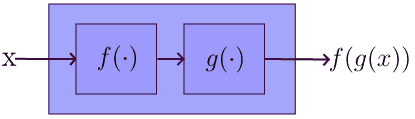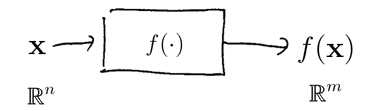# ML Wiki

## Function

In mathematics, a function $f(\cdot)$ is a set of pairs $x, f(x)$, where

• $x$ is input, $f(x)$ is output
• all possible inputs $x$ that a function can take is the domain of $f$
• all possible outputs $f(x)$ of a function $f(\cdot)$ is the range of $f$## Operations on Functions

### Composition

For two functions $f$ and $g$, composition is $f \circ g$

• $(f \circ g) (x) = f(g(x))$
• $g$ is applied first, then $f$For example,

• $\sqrt{1 - x^2}$
• $g = x^2$, it's inside
• $f(t) = \sqrt{1 - t}$, it's outsize

### Inverse

For $f$ its inverse is $f^{-1}$

• $x = f^{-1}(x)$ if $f(f^{-1}(x)) = x$
• $f^{-1}(\cdot)$ is a function that "undoes" $f(\cdot)$

## Single Variable Functions

That's the simplest type of functions: they have one input and one output

### Continuous Functions

• Functions are continuous is their Limits always exist
• otherwise functions are discontinuous
• these are important functions in Calculus

## Multi Variable Functions

These functions are more complex:

• they can have multiple inputs and multiple outputs
•## Function Spaces

Functions, like Vectors, can form Vector Spaces